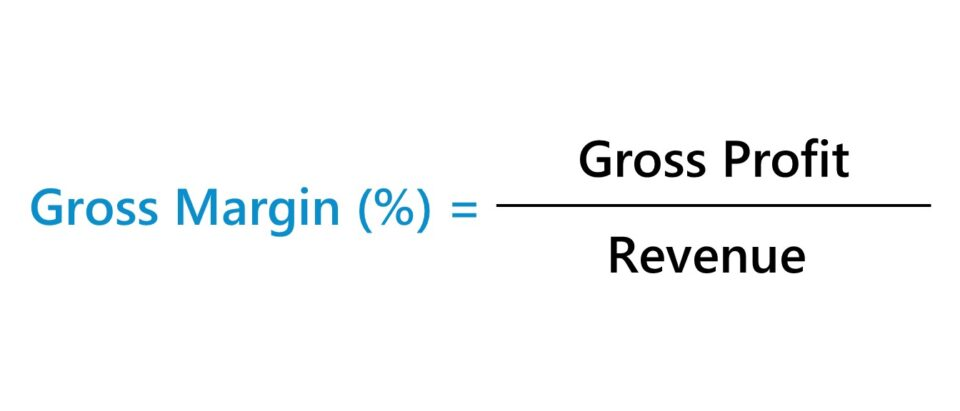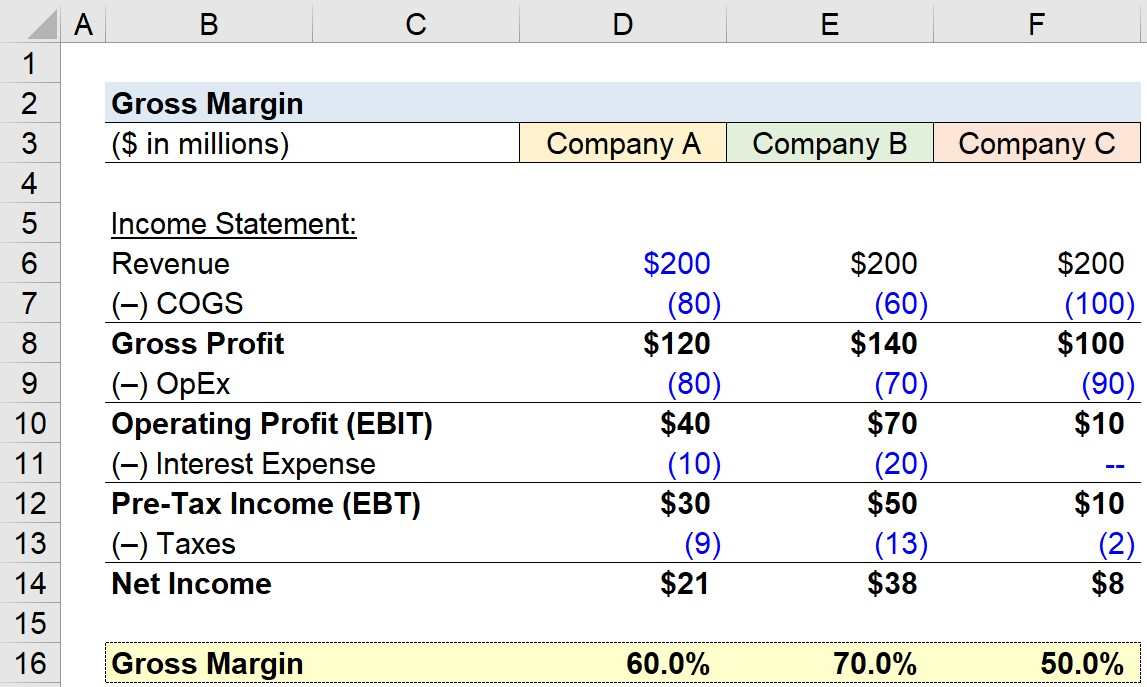Welcome to Wall Street Prep! Use code at checkout for 15% off.# Gross Margin

Guide to Understanding Gross Margin• The gross margin is a measure of profitability that compares a company’s gross profit to its revenue in the corresponding period.
• The formula to calculate gross margin divides a company’s gross profit in a given period by its revenue.
• The gross margin reflects the percentage of each dollar of revenue that a company retains as gross profit.
• The higher the gross margin ratio, the more revenue that remains upon subtracting cost of goods sold (COGS), which can be allocated towards operating expenses and non-operating costs.

## How to Calculate Gross Margin?

Calculating a company’s gross margin involves dividing its gross profit by the revenue in the matching period, which are both metrics found on the GAAP-based income statement.

The gross margin metric answers the following question, “How much in gross profits is kept for each dollar of revenue?”

• Gross Profit → The gross profit metric represents the remaining revenue after subtracting direct costs (COGS)
• Cost of Goods Sold (COGS) The direct costs incurred by a company that are directly tied to the production and delivery of specific goods and/or services (and are typically variable costs).

In accordance with GAAP accounting standards, the cost of goods sold (COGS) are matched to the associated revenues in the same time period, which is commonly known as the “matching principle”.

Common examples of costs recognized under the cost of goods sold (COGS) line item include the following:

• Direct Labor Costs
• Purchase of Materials (i.e. Inventory)

The process of calculating the gross profit margin is as follows:

1. Starting off, the net revenue and cost of goods sold (COGS) figures must be pulled from the income statement.
2. From that point, the gross profit is calculated by subtracting COGS from revenue.
3. To quantify the gross margin, the company’s gross profit must be divided by net revenue in the corresponding period.
4. The gross margin is expressed as a percentage, so the final step is to multiply the resulting figure by 100.

## Gross Margin Formula

The formula to calculate the gross profit margin is as follows.

Gross Margin (%) = Gross Profit ÷ Net Revenue
• Gross Profit = Net Revenue – Cost of Goods Sold (COGS)
• Net Revenue = Gross Revenue – Returns – Discounts

In order to express the metric in percentage form, the resulting decimal value figure must be multiplied by 100.

## Quick Gross Profit Margin Calculation Example

For example, if a company has generated \$10 million in revenue with \$8 million in COGS, the gross profit is \$2 million.

• Revenue = \$10 million
• Cost of Goods Sold (COGS) = \$8 million
• Gross Profit = \$10 million – \$8 million = \$2 million

Upon dividing the \$2 million in gross profit by the \$10 million in revenue and then multiplying by 100, we arrive at 20% as our gross profit margin.

• Gross Profit Margin (%) = \$2 million ÷ \$10 million = 0.20, or 20%

So, what does the 20% gross margin mean?

Since the gross margin ratio represents the percentage of a company’s revenue retained as gross profit, expressed on a per-dollar basis, the 20% gross margin implies that for each dollar of revenue generated, the company retains \$0.20, while \$0.80 is attributable to the incurred cost of goods sold (COGS).

## What is a Good Gross Profit Margin?

Interpreting a company’s gross margin as either “good” or “bad” depends substantially on the industry in which the company operates. For any comparisons to be useful, the companies must operate in the same or similar industry with available historical data dating back several years to get a better sense of the industry norm (and patterns).

• Higher Gross Margin (%) → For example, software companies have been known for having high gross margins, while clothing retailers have historically exhibited razor-thin gross margins and rely on volume to remain profitable. Service-based industries also tend to have higher gross margins since the COGS for such businesses are minimal (e.g. consulting firms).
• Lower Gross Margin (%) → In contrast, capital-intensive companies (e.g. manufacturing, industrials, retail) normally have lower gross margins due to margin erosion from the direct costs related to building up inventory, manufacturing products, etc. Nevertheless, such industries with significant direct costs can still consist of market leaders with consistent profits that retain meaningful market shares (e.g. airlines, transportation, retail).

As one would reasonably expect, higher gross margins are usually viewed in a positive light, as the potential for higher operating margins and net profit margins increases.

An accurate assessment of the gross profit metric depends, however, on understanding the industry dynamics and the company’s current business model.

## How to Increase Gross Profit Margin?

A couple of common methods for companies to improve their gross margin are the following:

1. Increase Average Selling Price (ASP) → Requires Pricing Power, Upselling, etc.
2. Purchase Inventory at Lower Prices → Requires Negotiating Leverage with Suppliers (e.g. High Order Volume/Frequency, Bulk Purchases, Branding)
3. Integrate Higher Margin Products/Services → Adding New Products/Services with Higher Gross Margins Could Lead to Improved Customer Retention and Cross-Selling Opportunities

## What is the Difference Between Gross Margin vs. Net Profit Margin?

• Gross Margin → The gross profit margin only accounts for direct costs (i.e. COGS), so the gross profit margin accounts for just one outflow of cash, which is the direct costs associated with the production of revenue. The gross profit margin, unlike the net margin, is largely unaffected by financing decisions or discretionary accounting policies such as useful life assumptions for purchases of PP&E or differences in the tax rate, making it better suited for peer comparisons since there is a far lower potential for manipulation via discretionary accounting decisions by management.
• Net Profit Margin → In contrast, the net profit margin accounts for all expenses, including operating expenses and non-operating expenses. The fact that net income is “levered” (i.e. post-debt) and flows solely to equity holders is one of the primary drawbacks to the net margin metric.

## Gross Margin Calculator

We’ll now move to a modeling exercise, which you can access by filling out the form below.Submitting...

## 1. Income Statement Assumptions

For our example modeling exercise, we’ll be calculating and comparing the gross profit margin of three companies, with each having different revenue and COGS assumptions.

We’ll start by listing out the revenue and cost of goods sold (COGS) assumptions for each company.

Income Statement – Company A

• Revenue = \$200m
• Cost of Goods Sold (COGS) = –\$80m

Income Statement – Company B

• Revenue = \$200m
• Cost of Goods Sold (COGS) = –\$60m

Income Statement – Company C

• Revenue = \$200m
• Cost of Goods Sold (COGS) = –\$100m

## 2. Gross Margin Calculation Example

Using these figures, we can calculate the gross profit for each company by subtracting COGS from revenue.

• Gross Profit, Company A: \$200m – \$80m = \$120m
• Gross Profit, Company B: \$200m – \$60m = \$140m
• Gross Profit, Company C: \$200m – \$100m = \$100m

Next, the gross profit of each company is divided by revenue to arrive at the gross profit margin metric.

• Gross Profit Margin (%), Company A: \$200m ÷ \$120m = 60.0%
• Gross Profit Margin (%), Company B: \$200m ÷ \$140m = 70.0%
• Gross Profit Margin (%), Company C: \$200m ÷ \$100m = 50.0%

## 3. Gross Profit Margin Ratio Analysis

Irrespective of the differences in operating expenses (OpEx), interest expenses, and tax rates among these companies, none of these differences are captured in the gross margin.

The gross margin isolates the profits only after COGS is factored in, which makes the metric more informative for peer group comparisons.

Since COGS were already taken into account, the remaining funds are available to be used to pay operating expenses (OpEx), interest expenses, and taxes.

For instance, the operating profit margin, which accounts for COGS and OpEx, is 20% for Company A, 35% for Company B, and 5% for Company C.

In conclusion, the gross margin should be used in conjunction with other metrics to fully understand the cost structure and business model of the company, as in the case of all profitability metrics.Step-by-Step Online Course

### Everything You Need To Master Financial Modeling

Enroll in The Premium Package: Learn Financial Statement Modeling, DCF, M&A, LBO and Comps. The same training program used at top investment banks.Inline FeedbacksLearn Financial Modeling Online

Everything you need to master financial and valuation modeling: 3-Statement Modeling, DCF, Comps, M&A and LBO.

X

The Wall Street Prep Quicklesson Series

7 Free Financial Modeling Lessons

Get instant access to video lessons taught by experienced investment bankers. Learn financial statement modeling, DCF, M&A, LBO, Comps and Excel shortcuts.